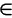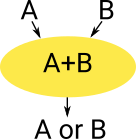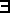# Maths - Types

We can look at types from a mathematical or a computing point of view.

### Types in Computing

In computing we think of type as representing some type of data structure, this determines:

• How the memory is allocated to store the type.
• Type Safety - Allows checks to be made on what operations can be done on the data to provide some level of program validation.
 See these pages for types as related to programming:

### Types in Mathematics

 Types were originally added to set theory to try to resolve some paradoxes that arose.Another way to look at it:

A type is the range of significance of a variable, that is, the concept of a vaiable is tied up with the concept of a type.

## Type Theorys

Here are some atomic mathematical types with some examples of terms that may inhabit those types:

Type Term
Boolean True
False

Natural Numbers 0,1,2...
Real Numbers 0.582
Set a

Type is a collection of terms having some common property.

Terms are values (or variables, or functions like the sum of other terms).

We can think of types as classifying values. If we know the type of something we have less information than knowing its value (and also its type). If we know a value without knowing its type, for example 0 (zero) we don't know if it is a natural number, or an integer, or a real number. Hence we don't know what operations might be valid to apply to it.

Types and terms are a similar (but different) concept to sets and elements. In both cases we think about it as a container relationship.

The ':' colon symbol is used to declare the type of a given term:

Type Term
M x:M typing declaration

If the type is not declared, it can be inferred in some circumstances.

We can have a multiple level hierarchy of types. For instance, the type 'M' in the above table may itself have a type of 'Type' ( M:Type ). However giving all higher order types the type of 'Type' could theoretically lead to circular references and hence paradoxes. Some theories allow these 'Type's to be numbered to enforce a hierarchy of universes and prevent any circular references. (see kinds)

## Martin-Löf Type Theorys

These are 'constructive' type theories also known as Intuitionistic type theories, that is, we can start with some atomic types as above and define compound types by building them up inductively.

There are a number of type theorys developed by Church, Martin-Löf, etc. Such as:

• STT - Simple Type Theory.
• PTT - Polymorphic Type Theory.
• DTT - Dependent Type Theory.

### Syntax

History: start with type theories with special types for natural numbers and truth values.

(see Lambek&Scott p129)

If A and B are valid types then we can define:

One Element Type Natural Numbers Container for elements of type A Product Truth Value
type notation 1 N PA A×B Ω
term (for type with intuitionistic predicate calculus) * 0,Sn {aA | φ(a)} <a,b> T,, ...
term (for type based on equality) * 0,Sn {aA | φ(a)} <a,b>

aα

a = a'

where:

• φ(a) is a term of type Ω (proof of type A ??, extension of power set ??)

#### Product and Sum Types

Product and sum types construct a type from 2 (or more) types (A and B).

 Product Type Has one constructor (formation) and multiple ways of destructuing (elimination) (projections).Sum Type Has multiple constructors but only one way to use it.### Sum, Product and Exponent

A type theory with Sum, Product and Exponent type is related to a Cartesian Closed Category (CCC).

A term may be represented by some variable such as x,y... or a compound term using the following:

If 'M' and 'N' are valid terms, then the following are also valid terms:

Type Term
M[x] We will use this notation to denote a term 'M' with multiple values depending on the value 'x'.
Sum A \/ B <M,N>

This is a union of the two types. An instance will contain an instance of only one of the types.

We can recover the contained types as follows:

• π1(M)
• π2(M)
Product A /\ B (M N) This contains both of the types (like tuples) . An instance will contain an instance of all of the types.
Exponent A->B λ x:M.N

This is a function from M to N.

where x is an instance of 'M' and the function returns an instance of 'N'

See lambda calculus.

Where:

• M,N are terms, that is a base type or a compound type made up as some combination of the above. That is, they are inductively defined.
• x is an instance of 'M'

Here are some more atomic types, this time denoted in a more category theory way:

Type Term
0 &bottom; empty type (initial)
1 &top; unit type (terminal)
2 True
False
boolean

### Constructors and Eliminators

List + pair function expression
constructor cons zero()
succ(n)
λ
type constructor     Pair   Atom
eliminator

car
cdr

function application

### Semantics

To put this in a slightly more formal setting see semantics page.

## Type Theory and Logic

There are various approaches to this:

• Curry-Howard correspondence.
• A logic is a logic over a type theory.

#### Curry-Howard

There is a correspondence between constructive (intuitionistic) logic and propositional logic.

Constructive Logic Type Theory
proposition type of its proofs A proposition is true iff we have a proof of that proposition
proof an object of that type

This does not work for more complicated type theorys and logics such as higher order logic.

#### Logic over a Type Theory

We treat logic as a meta-mathematics, that is a layer above the mathematics that allows us to apply axioms and rules to prove things about the mathematics.

### Type Theory and Information

Informally we sometimes think about 'information' when discussing types. for instance, does some particular operation loose or gain information. The concept of information was precisely defined by Claude Shannon in 1948. It is linked to the concept of 'entropy' which quantifies the amount of uncertainty involved. However something involving probabilities doesn't seem to be what's required here.

 So if we are trying to get an intuitive understanding of why the types/sets on the right are not isomorphic we need to see that the function shown can't be reversed. Intuitively we can see that this function 'looses information'. How can we make this more precise?More specifically, in type theory we have the 'computation rule' (beta reduction rule) and the 'Local completeness rule' (eta reduction rule) - see page here. We say that the 'beta reduction rule' ensures that information is not gained and the 'eta reduction rule' ensures that information is not lost.

One way to think about this is as a constructor as a producer of information (particle of information) and as an evaluator as a consumer (anti-information) which collide and annihilate in computation and local completeness rules.

## Comprehension

Any collection of elements of the same type may form an object of the next higher type.z'x[xz <-> Φ(x)]

## Concepts related to TypeBook Shop - Further reading.

Where I can, I have put links to Amazon for books that are relevant to the subject, click on the appropriate country flag to get more details of the book or to buy it from them.Computation and Reasoning - This book is about type theory. Although it is very technical it is aimed at computer scientists, so it has more discussion than a book aimed at pure mathematicians. It is especially useful for the coverage of dependant types.